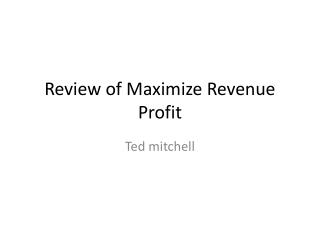Download PresentationReview of Maximize Revenue Profit

# Review of Maximize Revenue Profit

Download Presentation## Review of Maximize Revenue Profit

- - - - - - - - - - - - - - - - - - - - - - - - - - - E N D - - - - - - - - - - - - - - - - - - - - - - - - - - -
##### Presentation Transcript

1. Review of Maximize Revenue Profit Ted mitchell

2. Finding the Price to Maximize Revenue Ted Mitchell

3. Folklore and Beliefs • Under normal circumstances: If I increase my selling price, then I will increase the amount of stuff I’ll sell? • True or False

4. FolkLore and Beliefs • The optimal price in the new shoes game for maximizing profit is the price that maximizes revenue? • True or False

5. Folklore and Beliefs • Under normal circumstances: In new shoes simulation game If I decrease my optimal selling price for making profit, then I will increase my sales revenue. • True or False

6. Folklore and Beliefs • Cost based pricing is fair because it only bases along the increased cost of marking the product to the customer. • True or False

7. Folklore and Beliefs • Demand Based pricing is NOT fair because it shifts the whole amount of an increase in cost plus a bigger profit margin to the customer. • True or False

8. Higher Price Sells Fewer Units Quantity Sold Demand Equation Q = a - bP 100 ??? Price per Unit \$50 \$75 TJM

9. Get an estimate of a & b Quantity Demand Equation Q = 1,500 -5P a =1,500 X 500 X X X X X X X X X Price per Unit \$200 TJM

10. The Revenue Equation • Revenue, R = P xQ • Q = a - bP Substitute Q = (a-bP) • R = P(a - bP) • R = aP - bP2

11. \$5 Price Maximizes Revenue???R = 5,000P - 500P2 Revenue \$12,500 \$12,000 0 \$4 \$5 \$6 Price TJM

12. Find the optimal Price that Maximizes Revenue • Establish the Demand Function, Q = 5000 -500P • Establish the Revenue function • R = P x Q = P x (5000 -500P) = 5000P -500P2 • Find the first derivative wrt price • dR/dP = 5000 – 2(500)P = 5000 -1000P • Set the first derivative equal to zero and solve for P • 5000 – 1000P = 0 • P = 5000/1000 = \$5

13. The maximum revenue is • Revenue = P x Q = P x (5,000 – 500P) • Substitute optimal P = \$5 • Max revenue = 5,000P – 500P2 • Max revenue = 5(5,000 – 500(52)) • Max Revenue = 25,000 – 12,500 = \$12,500

14. The optimal price for max revenue • The Demand For Your Product Changes with The Price You Charge As • Q = 5000 - 500P

15. \$6 & \$4 Examples • The Demand For Your Product Changes with The Price You Charge AsQ = 5000 - 500P P = 5,000/2(500) = \$5

16. Price For Maximizing Profit by Ted Mitchell

17. Expand The Basic Profit Equation Z = PQ - VQ - F substitute Q = ƒ(P) = a - bP Z = P(a-bP) - V(a-bP) - F Z = aP - bP2 - aV + bPV - F

18. Revenue looks likeR = aP - bP2With zero costs Revenue = Profit Revenue = Profit 0 Price

19. Subtract Variable Costs from RevenueR - VQ - F = aP - bP2 - aV + bPV- F Revenue = Profit 0 P* Price P*

20. Example Exam Question • The Demand is estimated by market research to beQ = 5,000 – 500PThe variable cost per unit is, V = \$2The fixed cost for the period is, F = \$7,000What is the selling price that will maximize the Profit? • First build the Profit Equation, ZThe Revenue is R = P(a-bP2) = P(5,000-500P) • The Profit is Z = R – VQ – F • Z = P(5,000-500P) – 2(5,000-500P) – 7,000 • Z = 5,000P – 500P2 -10,000+1000P – 7,000

21. Example Exam Question • The Demand is estimated by market research to beQ = 5,000 – 500PThe variable cost per unit is, V = \$2The fixed cost for the period is, F = \$7,000What is the selling price that will maximize the Profit? • Second: Find the first derivative wrt P, • Z = 5,000P – 500P2 -10,000 + 1,000P – 7,000 • dZ/dP = 5,000 –2(500)P +1,000, set dZ/dP= 0 • 5,000 –2(500)P +1,000 = 0 , solve for P • –2(500)P = -5,000 -1,000 = P = 6,000/1,000 = \$6

22. Revenue Profit \$ \$5 0 \$6 Price

23. There is a General Solution for Finding Optimal Price for Max Profit • Establish the Profit equation • Z = aP - bP2 - aV + bPV – F • Find the first derivative of the profit equation • dZ/dP = a – 2bP – bV • Set the first derivative equal to zero • dZ/dP = a – 2bP – bV = 0 • Solve for the optimal price • P = a/2b + bV/2b = a/2b + V/2b

24. The Price That Maximizes Profit Consider Market Potential Consider Your Variable Costs Consider The Customer’s Sensitivity to Price Changes

25. The Price That Maximizes Profit P = (Price that maximizes revenue) + (Half of the Variable Cost)